# Spectrogram¶

‘audio.spectrogram’ module within the ketos library.

This module provides utilities to work with spectrograms.

Spectrograms are two-dimensional visual representations of sound waves, in which time is shown along the horizontal axis, frequency along the vertical axis, and color is used to indicate the sound amplitude. Read more on Wikipedia: https://en.wikipedia.org/wiki/Spectrogram

The module contains the parent class Spectrogram, and four child classes (MagSpectrogram, PowerSpectrogram, MelSpectrogram, CQTSpectrogram), which inherit methods and attributes from the parent class.

Note, however, that not all methods (e.g. crop) work for all child classes. See the documentation of the individual methods for further details.

Contents:

Spectrogram class: MagSpectrogram class: PowerSpectrogram class: MelSpectrogram class: CQTSpectrogram class

class `ketos.audio.spectrogram.``CQTSpectrogram`(data, time_res, bins_per_oct, freq_min, window_func=None, filename=None, offset=0, label=None, annot=None, transforms=None, transform_log=None, waveform_transform_log=None, **kwargs)[source]

Magnitude Spectrogram computed from Constant Q Transform (CQT).

Args:
image: 2d or 3d numpy array

Spectrogram pixel values.

time_res: float

Time resolution in seconds (corresponds to the bin size used on the time axis)

freq_min: float

Lower value of the frequency axis in Hz

bins_per_oct: int

Number of bins per octave

window_func: str

Window function used for computing the spectrogram

filename: str or list(str)

Name of the source audio file, if available.

offset: float or array-like

Position in seconds of the left edge of the spectrogram within the source audio file, if available.

label: int

Spectrogram label. Optional

annot: AnnotationHandler

AnnotationHandler object. Optional

transforms: list(dict)

List of dictionaries, where each dictionary specifies the name of a transformation to be applied to the spectrogram. For example, {“name”:”normalize”, “mean”:0.5, “std”:1.0}

transform_log: list(dict)

List of transforms that have been applied to this spectrogram

waveform_transform_log: list(dict)

List of transforms that have been applied to the waveform before generating this spectrogram

Attrs:
window_func: str

Window function.

`bins_per_octave`()[source]

Get no. bins per octave.

Returns:
: int

No. bins per octave.

classmethod `empty`()[source]

Creates an empty CQTSpectrogram object

classmethod `from_wav`(path, step, bins_per_oct, freq_min=1, freq_max=None, channel=0, rate=None, window_func='hann', offset=0, duration=None, resample_method='scipy', id=None, normalize_wav=False, transforms=None, waveform_transforms=None, **kwargs)[source]

Create CQT spectrogram directly from wav file.

The arguments offset and duration can be used to select a segment of the audio file.

Note that values specified for the arguments window, step, offset, and duration may all be subject to slight adjustments to ensure that the selected portion corresponds to an integer number of window frames, and that the window and step sizes correspond to an integer number of samples.

Args:
path: str

Complete path to wav file

step: float

Step size in seconds

bins_per_oct: int

Number of bins per octave

freq_min: float

Minimum frequency in Hz. Default is 1 Hz.

freq_max: float

Maximum frequency in Hz If None, it is set half the sampling rate.

channel: int

Channel to read from. Only relevant for stereo recordings

rate: float

Desired sampling rate in Hz. If None, the original sampling rate will be used

window_func: str
Window function (optional). Select between
• bartlett

• blackman

• hamming (default)

• hanning

offset: float

Start time of spectrogram in seconds, relative the start of the wav file.

duration: float

Length of spectrogrma in seconds.

resample_method: str
Resampling method. Only relevant if rate is specified. Options are
• kaiser_best

• kaiser_fast

• scipy (default)

• polyphase

See https://librosa.github.io/librosa/generated/librosa.core.resample.html for details on the individual methods.

id: str

Unique identifier (optional). If None, the filename will be used.

normalize_wav: bool

Normalize the waveform to have a mean of zero (mean=0) and a standard deviation of unity (std=1) before computing the spectrogram. Default is False.

transforms: list(dict)

List of dictionaries, where each dictionary specifies the name of a transformation to be applied to the spectrogram. For example, {“name”:”normalize”, “mean”:0.5, “std”:1.0}

waveform_transforms: list(dict)

List of dictionaries, where each dictionary specifies the name of a transformation to be applied to the waveform before generating the spectrogram. For example, {“name”:”add_gaussian_noise”, “sigma”:0.5}

Returns:
: CQTSpectrogram

CQT spectrogram

Example:
```>>> # load spectrogram from wav file
>>> from ketos.audio.spectrogram import CQTSpectrogram
>>> spec = CQTSpectrogram.from_wav('ketos/tests/assets/grunt1.wav', step=0.01, freq_min=10, freq_max=800, bins_per_oct=16)
>>> # show
>>> fig = spec.plot()
>>> fig.savefig("ketos/tests/assets/tmp/cqt_grunt1.png")
>>> plt.close(fig)
```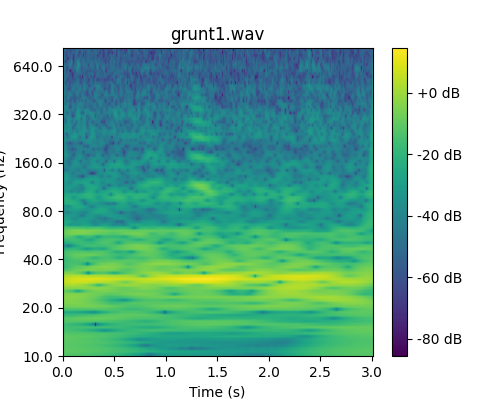classmethod `from_waveform`(audio, step, bins_per_oct, freq_min=1, freq_max=None, window_func='hann', transforms=None, **kwargs)[source]

Magnitude Spectrogram computed from Constant Q Transform (CQT) using the librosa implementation:

https://librosa.github.io/librosa/generated/librosa.core.cqt.html

The frequency axis of a CQT spectrogram is essentially a logarithmic axis with base 2. It is characterized by an integer number of bins per octave (an octave being a doubling of the frequency.)

For further details, see `audio.audio.cqt()`.

Args:
audio: Waveform

Audio signal

step: float

Step size in seconds

bins_per_oct: int

Number of bins per octave

freq_min: float

Minimum frequency in Hz. Default is 1 Hz.

freq_max: float

Maximum frequency in Hz If None, it is set half the sampling rate.

window_func: str
Window function (optional). Select between
• bartlett

• blackman

• hamming

• hanning (default)

transforms: list(dict)

List of dictionaries, where each dictionary specifies the name of a transformation to be applied to the spectrogram. For example, {“name”:”normalize”, “mean”:0.5, “std”:1.0}

Returns:
spec: CQTSpectrogram

CQT spectrogram

`get_attrs`()[source]

Get scalar attributes

`plot`(id=0, show_annot=False, figsize=5, 4, cmap='viridis', label_in_title=True)[source]

Plot the spectrogram with proper axes ranges and labels.

Optionally, also display annotations as boxes superimposed on the spectrogram.

The colormaps available can be seen here: https://matplotlib.org/3.1.0/tutorials/colors/colormaps.html

Note: The resulting figure can be shown (fig.show()) or saved (fig.savefig(file_name))

Args:
id: int

Spectrogram to be plotted. Only relevant if the spectrogram object contains multiple, stacked spectrograms.

show_annot: bool

Display annotations

figsize: tuple

Figure size

cmap: string

The colormap to be used

label_in_title: bool

Include label (if available) in figure title

Returns:
fig: matplotlib.figure.Figure

A figure object.

class `ketos.audio.spectrogram.``MagSpectrogram`(data, time_res, freq_min, freq_res, window_func=None, filename=None, offset=0, label=None, annot=None, transforms=None, transform_log=None, waveform_transform_log=None, **kwargs)[source]

Magnitude Spectrogram.

Args:
data: 2d or 3d numpy array

Spectrogram pixel values.

time_res: float

Time resolution in seconds (corresponds to the bin size used on the time axis)

freq_min: float

Lower value of the frequency axis in Hz

freq_res: float

Frequency resolution in Hz (corresponds to the bin size used on the frequency axis)

window_func: str

Window function used for computing the spectrogram

filename: str or list(str)

Name of the source audio file, if available.

offset: float or array-like

Position in seconds of the left edge of the spectrogram within the source audio file, if available.

label: int

Spectrogram label. Optional

annot: AnnotationHandler

AnnotationHandler object. Optional

transforms: list(dict)

List of dictionaries, where each dictionary specifies the name of a transformation to be applied to the spectrogram. For example, {“name”:”normalize”, “mean”:0.5, “std”:1.0}

transform_log: list(dict)

List of transforms that have been applied to this spectrogram

waveform_transform_log: list(dict)

List of transforms that have been applied to the waveform before generating this spectrogram

Attrs:
window_func: str

Window function.

classmethod `empty`()[source]

Creates an empty MagSpectrogram object

`freq_res`()[source]

Get frequency resolution in Hz.

Returns:
: float

Frequency resolution in Hz

classmethod `from_wav`(path, window, step, channel=0, rate=None, window_func='hamming', offset=0, duration=None, resample_method='scipy', freq_min=None, freq_max=None, id=None, normalize_wav=False, transforms=None, waveform_transforms=None, **kwargs)[source]

Create magnitude spectrogram directly from wav file.

The arguments offset and duration can be used to select a portion of the wav file.

Note that values specified for the arguments window, step, offset, and duration may all be subject to slight adjustments to ensure that the selected portion corresponds to an integer number of window frames, and that the window and step sizes correspond to an integer number of samples.

Args:
path: str

Path to wav file

window: float

Window size in seconds

step: float

Step size in seconds

channel: int

Channel to read from. Only relevant for stereo recordings

rate: float

Desired sampling rate in Hz. If None, the original sampling rate will be used

window_func: str
Window function (optional). Select between
• bartlett

• blackman

• hamming (default)

• hanning

offset: float

Start time of spectrogram in seconds, relative the start of the wav file.

duration: float

Length of spectrogram in seconds.

resample_method: str
Resampling method. Only relevant if rate is specified. Options are
• kaiser_best

• kaiser_fast

• scipy (default)

• polyphase

See https://librosa.github.io/librosa/generated/librosa.core.resample.html for details on the individual methods.

freq_min: float

Lower frequency in Hz.

freq_max: str or float

Upper frequency in Hz.

id: str

Unique identifier (optional). If None, the filename will be used.

normalize_wav: bool

Normalize the waveform to have a mean of zero (mean=0) and a standard deviation of unity (std=1) before computing the spectrogram. Default is False.

transforms: list(dict)

List of dictionaries, where each dictionary specifies the name of a transformation to be applied to the spectrogram. For example, {“name”:”normalize”, “mean”:0.5, “std”:1.0}

waveform_transforms: list(dict)

List of dictionaries, where each dictionary specifies the name of a transformation to be applied to the waveform before generating the spectrogram. For example, {“name”:”add_gaussian_noise”, “sigma”:0.5}

Returns:
: MagSpectrogram

Magnitude spectrogram

Example:
```>>> # load spectrogram from wav file
>>> from ketos.audio.spectrogram import MagSpectrogram
>>> spec = MagSpectrogram.from_wav('ketos/tests/assets/grunt1.wav', window=0.2, step=0.01)
>>> # crop frequency
>>> spec = spec.crop(freq_min=50, freq_max=800)
>>> # show
>>> fig = spec.plot()
>>> fig.savefig("ketos/tests/assets/tmp/spec_grunt1.png")
>>> plt.close(fig)
```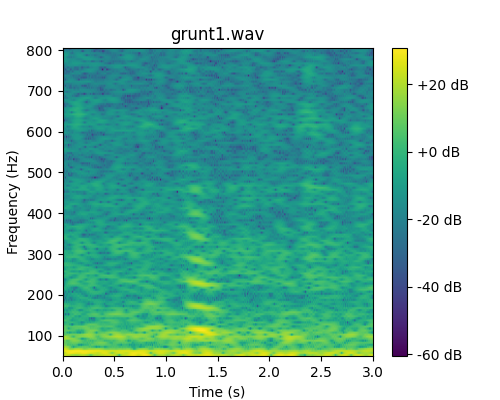classmethod `from_waveform`(audio, window=None, step=None, seg_args=None, window_func='hamming', freq_min=None, freq_max=None, transforms=None, **kwargs)[source]

Create a Magnitude Spectrogram from an `audio_signal.Waveform` by computing the Short Time Fourier Transform (STFT).

Args:
audio: Waveform

Audio signal

window: float

Window length in seconds

step: float

Step size in seconds

seg_args: dict

Input arguments used for evaluating `audio.audio.segment_args()`. Optional. If specified, the arguments window and step are ignored.

window_func: str
Window function (optional). Select between
• bartlett

• blackman

• hamming (default)

• hanning

freq_min: float

Lower frequency in Hz.

freq_max: str or float

Upper frequency in Hz.

transforms: list(dict)

List of dictionaries, where each dictionary specifies the name of a transformation to be applied to the spectrogram. For example, {“name”:”normalize”, “mean”:0.5, “std”:1.0}

Returns:
spec: MagSpectrogram

Magnitude spectrogram

`get_attrs`()[source]

Get scalar attributes

`recover_waveform`(num_iters=25, phase_angle=0)[source]

Estimate audio signal from magnitude spectrogram.

Uses `audio.audio.spec2wave()`.

Args:
num_iters:

Number of iterations to perform.

phase_angle:

Initial condition for phase.

Returns:
: Waveform

Audio signal

class `ketos.audio.spectrogram.``MelSpectrogram`(data, filter_banks, time_res, freq_min, freq_max, window_func=None, filename=None, offset=0, label=None, annot=None, transforms=None, transform_log=None, waveform_transform_log=None, **kwargs)[source]

Mel Spectrogram.

Args:
data: 2d or 3d numpy array

Mel spectrogram pixel values.

filter_banks: numpy.array

Filter banks

time_res: float

Time resolution in seconds (corresponds to the bin size used on the time axis)

freq_min: float

Lower value of the frequency axis in Hz

freq_max: float

Upper value of the frequency axis in Hz

window_func: str

Window function used for computing the spectrogram

filename: str or list(str)

Name of the source audio file, if available.

offset: float or array-like

Position in seconds of the left edge of the spectrogram within the source audio file, if available.

label: int

Spectrogram label. Optional

annot: AnnotationHandler

AnnotationHandler object. Optional

transforms: list(dict)

List of dictionaries, where each dictionary specifies the name of a transformation to be applied to the spectrogram. For example, {“name”:”normalize”, “mean”:0.5, “std”:1.0}

transform_log: list(dict)

List of transforms that have been applied to this spectrogram

waveform_transform_log: list(dict)

List of transforms that have been applied to the waveform before generating this spectrogram

Attrs:
window_func: str

Window function.

filter_banks: numpy.array

Filter banks

classmethod `empty`()[source]

Creates an empty MelSpectrogram object

classmethod `from_wav`(path, window, step, channel=0, rate=None, window_func='hamming', num_filters=40, num_ceps=20, cep_lifter=20, offset=0, duration=None, resample_method='scipy', id=None, normalize_wav=False, transforms=None, waveform_transforms=None, **kwargs)[source]

Create Mel spectrogram directly from wav file.

The arguments offset and duration can be used to select a portion of the wav file.

Note that values specified for the arguments window, step, offset, and duration may all be subject to slight adjustments to ensure that the selected portion corresponds to an integer number of window frames, and that the window and step sizes correspond to an integer number of samples.

Args:
path: str

Path to wav file

window: float

Window size in seconds

step: float

Step size in seconds

channel: int

Channel to read from. Only relevant for stereo recordings

rate: float

Desired sampling rate in Hz. If None, the original sampling rate will be used

window_func: str
Window function (optional). Select between
• bartlett

• blackman

• hamming (default)

• hanning

num_filters: int

The number of filters in the filter bank.

num_ceps: int

The number of Mel-frequency cepstrums.

cep_lifters: int

The number of cepstum filters.

offset: float

Start time of spectrogram in seconds, relative the start of the wav file.

duration: float

Length of spectrogrma in seconds.

resample_method: str
Resampling method. Only relevant if rate is specified. Options are
• kaiser_best

• kaiser_fast

• scipy (default)

• polyphase

See https://librosa.github.io/librosa/generated/librosa.core.resample.html for details on the individual methods.

id: str

Unique identifier (optional). If None, the filename will be used.

normalize_wav: bool

Normalize the waveform to have a mean of zero (mean=0) and a standard deviation of unity (std=1) before computing the spectrogram. Default is False.

transforms: list(dict)

List of dictionaries, where each dictionary specifies the name of a transformation to be applied to the spectrogram. For example, {“name”:”normalize”, “mean”:0.5, “std”:1.0}

waveform_transforms: list(dict)

List of dictionaries, where each dictionary specifies the name of a transformation to be applied to the waveform before generating the spectrogram. For example, {“name”:”add_gaussian_noise”, “sigma”:0.5}

Returns:
spec: MelSpectrogram

Mel spectrogram

Example:
```>>> # load spectrogram from wav file
>>> from ketos.audio.spectrogram import MelSpectrogram
>>> spec = MelSpectrogram.from_wav('ketos/tests/assets/grunt1.wav', window=0.2, step=0.01)
>>> # crop frequency
>>> spec = spec.crop(freq_min=50, freq_max=800)
>>> # show
>>> fig = spec.plot()
>>> fig.savefig("ketos/tests/assets/tmp/mel_grunt1.png")
>>> plt.close(fig)
```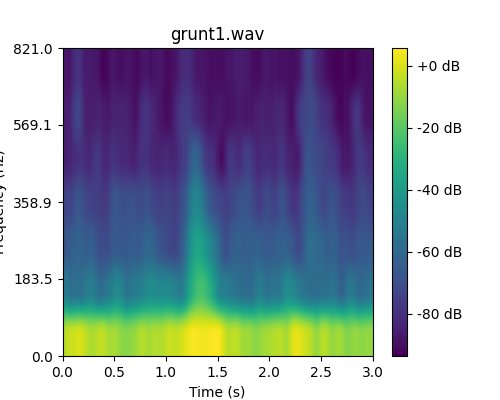classmethod `from_waveform`(audio, window=None, step=None, seg_args=None, window_func='hamming', num_filters=40, num_ceps=20, cep_lifter=20, transforms=None, **kwargs)[source]

Creates a Mel Spectrogram from an `audio_signal.Waveform`.

Args:
audio: Waveform

Audio signal

window: float

Window length in seconds

step: float

Step size in seconds

seg_args: dict

Input arguments used for evaluating `audio.audio.segment_args()`. Optional. If specified, the arguments window and step are ignored.

window_func: str
Window function (optional). Select between
• bartlett

• blackman

• hamming (default)

• hanning

num_filters: int

The number of filters in the filter bank.

num_ceps: int

The number of Mel-frequency cepstrums.

cep_lifters: int

The number of cepstum filters.

transforms: list(dict)

List of dictionaries, where each dictionary specifies the name of a transformation to be applied to the spectrogram. For example, {“name”:”normalize”, “mean”:0.5, “std”:1.0}

Returns:
: MelSpectrogram

Mel spectrogram

`get_attrs`()[source]

Get scalar attributes

`plot`(filter_bank=False, figsize=5, 4, cmap='viridis')[source]

Plot the spectrogram with proper axes ranges and labels.

The colormaps available can be seen here: https://matplotlib.org/3.1.0/tutorials/colors/colormaps.html

Note: The resulting figure can be shown (fig.show()) or saved (fig.savefig(file_name))

TODO: Check implementation for filter_bank=True

Args:
filter_bank: bool

If True, plot the filter banks if True. If False (default), print the mel spectrogram.

figsize: tuple

Figure size

cmap: string

The colormap to be used

Returns:
fig: matplotlib.figure.Figure

A figure object.

class `ketos.audio.spectrogram.``PowerSpectrogram`(data, time_res, freq_min, freq_res, window_func=None, filename=None, offset=0, label=None, annot=None, transforms=None, transform_log=None, waveform_transform_log=None, **kwargs)[source]

Power Spectrogram.

Args:
data: 2d or 3d numpy array

Spectrogram pixel values.

time_res: float

Time resolution in seconds (corresponds to the bin size used on the time axis)

freq_min: float

Lower value of the frequency axis in Hz

freq_res: float

Frequency resolution in Hz (corresponds to the bin size used on the frequency axis)

window_func: str

Window function used for computing the spectrogram

filename: str or list(str)

Name of the source audio file, if available.

offset: float or array-like

Position in seconds of the left edge of the spectrogram within the source audio file, if available.

label: int

Spectrogram label. Optional

annot: AnnotationHandler

AnnotationHandler object. Optional

transforms: list(dict)

List of dictionaries, where each dictionary specifies the name of a transformation to be applied to the spectrogram. For example, {“name”:”normalize”, “mean”:0.5, “std”:1.0}

transform_log: list(dict)

List of transforms that have been applied to this spectrogram

waveform_transform_log: list(dict)

List of transforms that have been applied to the waveform before generating this spectrogram

Attrs:
window_func: str

Window function.

classmethod `empty`()[source]

Creates an empty PowerSpectrogram object

`freq_res`()[source]

Get frequency resolution in Hz.

Returns:
: float

Frequency resolution in Hz

classmethod `from_wav`(path, window, step, channel=0, rate=None, window_func='hamming', offset=0, duration=None, resample_method='scipy', freq_min=None, freq_max=None, id=None, normalize_wav=False, transforms=None, waveform_transforms=None, **kwargs)[source]

Create power spectrogram directly from wav file.

The arguments offset and duration can be used to select a portion of the wav file.

Note that values specified for the arguments window, step, offset, and duration may all be subject to slight adjustments to ensure that the selected portion corresponds to an integer number of window frames, and that the window and step sizes correspond to an integer number of samples.

Args:
path: str

Path to wav file

window: float

Window size in seconds

step: float

Step size in seconds

channel: int

Channel to read from. Only relevant for stereo recordings

rate: float

Desired sampling rate in Hz. If None, the original sampling rate will be used

window_func: str
Window function (optional). Select between
• bartlett

• blackman

• hamming (default)

• hanning

offset: float

Start time of spectrogram in seconds, relative the start of the wav file.

duration: float

Length of spectrogrma in seconds.

resample_method: str
Resampling method. Only relevant if rate is specified. Options are
• kaiser_best

• kaiser_fast

• scipy (default)

• polyphase

See https://librosa.github.io/librosa/generated/librosa.core.resample.html for details on the individual methods.

freq_min: float

Lower frequency in Hz.

freq_max: str or float

Upper frequency in Hz.

id: str

Unique identifier (optional). If None, the filename will be used.

normalize_wav: bool

Normalize the waveform to have a mean of zero (mean=0) and a standard deviation of unity (std=1) before computing the spectrogram. Default is False.

transforms: list(dict)

List of dictionaries, where each dictionary specifies the name of a transformation to be applied to the spectrogram. For example, {“name”:”normalize”, “mean”:0.5, “std”:1.0}

waveform_transforms: list(dict)

List of dictionaries, where each dictionary specifies the name of a transformation to be applied to the waveform before generating the spectrogram. For example, {“name”:”add_gaussian_noise”, “sigma”:0.5}

Returns:
spec: MagSpectrogram

Magnitude spectrogram

Example:
```>>> # load spectrogram from wav file
>>> from ketos.audio.spectrogram import MagSpectrogram
>>> spec = MagSpectrogram.from_wav('ketos/tests/assets/grunt1.wav', window=0.2, step=0.01)
>>> # crop frequency
>>> spec = spec.crop(freq_min=50, freq_max=800)
>>> # show
>>> fig = spec.plot()
>>> fig.savefig("ketos/tests/assets/tmp/spec_grunt1.png")
>>> plt.close(fig)
```classmethod `from_waveform`(audio, window=None, step=None, seg_args=None, window_func='hamming', freq_min=None, freq_max=None, transforms=None, **kwargs)[source]

Create a Power Spectrogram from an `audio_signal.Waveform` by computing the Short Time Fourier Transform (STFT).

Args:
audio: Waveform

Audio signal

window: float

Window length in seconds

step: float

Step size in seconds

seg_args: dict

Input arguments used for evaluating `audio.audio.segment_args()`. Optional. If specified, the arguments window and step are ignored.

window_func: str
Window function (optional). Select between
• bartlett

• blackman

• hamming (default)

• hanning

freq_min: float

Lower frequency in Hz.

freq_max: str or float

Upper frequency in Hz.

transforms: list(dict)

List of dictionaries, where each dictionary specifies the name of a transformation to be applied to the spectrogram. For example, {“name”:”normalize”, “mean”:0.5, “std”:1.0}

Returns:
: MagSpectrogram

Magnitude spectrogram

`get_attrs`()[source]

Get scalar attributes

class `ketos.audio.spectrogram.``Spectrogram`(data, time_res, spec_type, freq_ax, filename=None, offset=0, label=None, annot=None, transforms=None, transform_log=None, waveform_transform_log=None, **kwargs)[source]

Spectrogram.

Parent class for MagSpectrogram, PowerSpectrogram, MelSpectrogram, and CQTSpectrogram.

The Spectrogram class stores the spectrogram pixel values in a 2d numpy array, where the first axis (0) is the time dimension and the second axis (1) is the frequency dimensions.

The Spectrogram class can also store a stack of multiple, identical-size, spectrograms in a 3d numpy array with the last axis (3) representing the multiple instances.

Args:
image: 2d or 3d numpy array

Spectrogram pixel values.

time_res: float

Time resolution in seconds (corresponds to the bin size used on the time axis)

spec_type: str
Spectrogram type. Options include,
• ‘Mag’: Magnitude spectrogram

• ‘Pow’: Power spectrogram

• ‘Mel’: Mel spectrogram

• ‘CQT’: CQT spectrogram

freq_ax: LinearAxis or Log2Axis

Axis object for the frequency dimension

filename: str or list(str)

Name of the source audio file, if available.

offset: float or array-like

Position in seconds of the left edge of the spectrogram within the source audio file, if available.

label: int

Spectrogram label. Optional

annot: AnnotationHandler

AnnotationHandler object. Optional

transforms: list(dict)

List of dictionaries, where each dictionary specifies the name of a transformation to be applied to the spectrogram. For example, {“name”:”normalize”, “mean”:0.5, “std”:1.0}

transform_log: list(dict)

List of transforms that have been applied to this spectrogram

waveform_transform_log: list(dict)

List of transforms that have been applied to the waveform before generating this spectrogram

Attributes:
image: 2d or 3d numpy array

Spectrogram pixel values.

time_ax: LinearAxis

Axis object for the time dimension

freq_ax: LinearAxis or Log2Axis

Axis object for the frequency dimension

type: str
Spectrogram type. Options include,
• ‘Mag’: Magnitude spectrogram

• ‘Pow’: Power spectrogram

• ‘Mel’: Mel spectrogram

• ‘CQT’: CQT spectrogram

filename: str or list(str)

Name of the source audio file.

offset: float or array-like

Position in seconds of the left edge of the spectrogram within the source audio file.

label: int

Spectrogram label.

annot: AnnotationHandler

AnnotationHandler object.

transform_log: list(dict)

List of transforms that have been applied to this spectrogram

waveform_transform_log: list(dict)

List of transforms that have been applied to the waveform before generating this spectrogram

`add`(spec, offset=0, scale=1, make_copy=False)[source]

Add another spectrogram on top of this spectrogram.

The spectrograms must be of the same type, and share the same time resolution.

The spectrograms must have consistent frequency axes. For linear frequency axes, this implies having the same resolution; for logarithmic axes with base 2, this implies having the same number of bins per octave minimum values that differ by a factor ofwhereis the number of bins per octave andis any integer. No check is made for the consistency of the frequency axes.

Note that the attributes filename, offset, and label of the spectrogram that is being added are lost.

The sum spectrogram has the same dimensions (time x frequency) as the original spectrogram.

Args:
spec: Spectrogram

Spectrogram to be added

offset: float

Shift the spectrograms that is being added by this many seconds relative to the original spectrogram.

scale: float

Scaling factor applied to spectrogram that is added

make_copy: bool

Make copies of both spectrograms so as to leave the original instances unchanged.

Returns:
: Spectrogram

Sum spectrogram

`blur`(sigma_time, sigma_freq=0)[source]

Blur the spectrogram using a Gaussian filter.

Note that the spectrogram frequency axis must be linear if sigma_freq > 0.

This uses the Gaussian filter method from the scipy.ndimage package:

Args:
sigma_time: float

Gaussian kernel standard deviation along time axis in seconds. Must be strictly positive.

sigma_freq: float

Gaussian kernel standard deviation along frequency axis in Hz.

Example:
```>>> from ketos.audio.spectrogram import Spectrogram
>>> from ketos.audio.waveform import Waveform
>>> import matplotlib.pyplot as plt
>>> # create audio signal
>>> s = Waveform.morlet(rate=1000, frequency=300, width=1)
>>> # create spectrogram
>>> spec = MagSpectrogram.from_waveform(s, window=0.2, step=0.05)
>>> # show image
>>> fig = spec.plot()
>>> plt.close(fig)
>>> # apply very small amount (0.01 sec) of horizontal blur
>>> # and significant amount of vertical blur (30 Hz)
>>> spec.blur(sigma_time=0.01, sigma_freq=30)
>>> # show blurred image
>>> fig = spec.plot()
>>> plt.close(fig)
```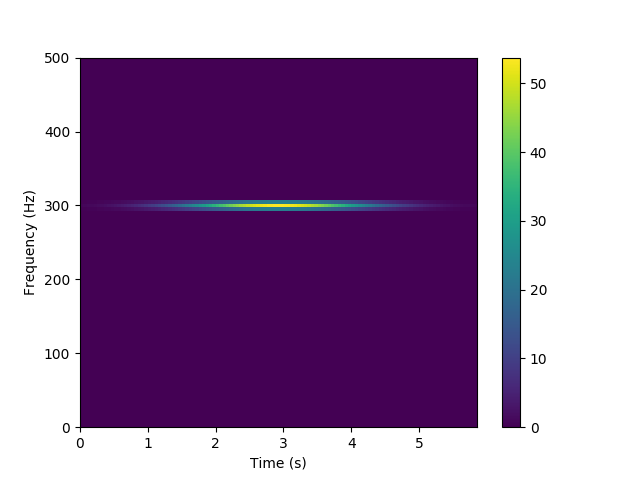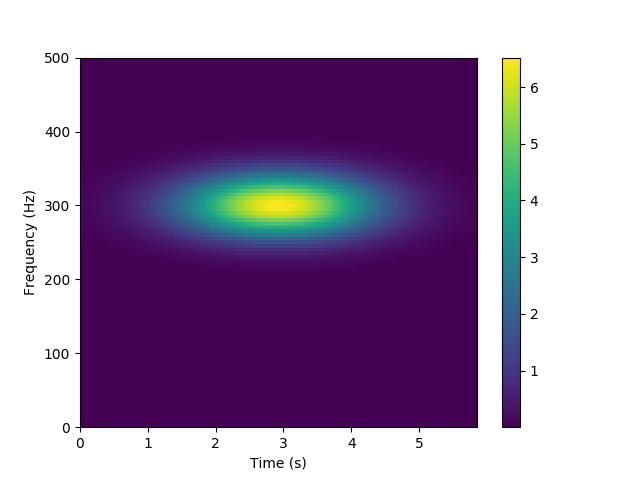`crop`(start=None, end=None, length=None, freq_min=None, freq_max=None, height=None, make_copy=False)[source]

Crop spectogram along time axis, frequency axis, or both.

Args:
start: float

Start time in seconds, measured from the left edge of spectrogram.

end: float

End time in seconds, measured from the left edge of spectrogram.

length: int

Horizontal size of the cropped image (number of pixels). If provided, the end argument is ignored.

freq_min: float

Lower frequency in Hz.

freq_max: str or float

Upper frequency in Hz.

height: int

Vertical size of the cropped image (number of pixels). If provided, the freq_max argument is ignored.

make_copy: bool

Return a cropped copy of the spectrogra. Leaves the present instance unaffected. Default is False.

Returns:
spec: Spectrogram

Cropped spectrogram

Examples:
```>>> import numpy as np
>>> import matplotlib.pyplot as plt
>>> from ketos.audio.spectrogram import Spectrogram
>>> from ketos.audio.utils.axis import LinearAxis
>>> # Create a spectrogram with shape (20,30), time resolution of
>>> # 0.5 s, random pixel values, and a linear frequency axis from
>>> # 0 to 300 Hz,
>>> ax = LinearAxis(bins=30, extent=(0.,300.), label='Frequency (Hz)')
>>> img = np.random.rand(20,30)
>>> spec = Spectrogram(data=img, time_res=0.5, spec_type='Mag', freq_ax=ax)
>>> # Draw the spectrogram
>>> fig = spec.plot()
>>> fig.savefig("ketos/tests/assets/tmp/spec_orig.png")
>>> plt.close(fig)
```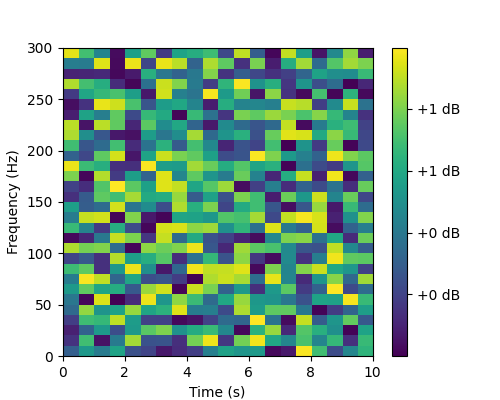```>>> # Crop the spectrogram along time axis
>>> spec1 = spec.crop(start=2.0, end=4.2, make_copy=True)
>>> # Draw the spectrogram
>>> fig = spec.plot()
>>> fig.savefig("ketos/tests/assets/tmp/spec_cropped.png")
>>> plt.close(fig)
````enhance_signal`(enhancement=1.0)[source]

Enhance the contrast between regions of high and low intensity.

See `audio.image.enhance_image()` for implementation details.

Args:
enhancement: float

Parameter determining the amount of enhancement.

`freq_max`()[source]

Get spectrogram maximum frequency in Hz.

Returns:
: float

Frequency in Hz

`freq_min`()[source]

Get spectrogram minimum frequency in Hz.

Returns:
: float

Frequency in Hz

`get_attrs`()[source]

Get scalar attributes

`plot`(id=0, show_annot=False, figsize=5, 4, cmap='viridis', label_in_title=True)[source]

Plot the spectrogram with proper axes ranges and labels.

Optionally, also display annotations as boxes superimposed on the spectrogram.

The colormaps available can be seen here: https://matplotlib.org/3.1.0/tutorials/colors/colormaps.html

Note: The resulting figure can be shown (fig.show()) or saved (fig.savefig(file_name))

Args:
id: int

Spectrogram to be plotted. Only relevant if the spectrogram object contains multiple, stacked spectrograms.

show_annot: bool

Display annotations

figsize: tuple

Figure size

cmap: string

The colormap to be used

label_in_title: bool

Include label (if available) in figure title

Returns:

fig: matplotlib.figure.Figure A figure object.

Example:
```>>> from ketos.audio.spectrogram import MagSpectrogram
>>> # load spectrogram
>>> spec = MagSpectrogram.from_wav('ketos/tests/assets/grunt1.wav', window=0.2, step=0.02)
>>> # add an annotation
>>> spec.annotate(start=1.2, end=1.6, freq_min=70, freq_max=600, label=1)
>>> # keep only frequencies below 800 Hz
>>> spec = spec.crop(freq_max=800)
>>> # show spectrogram with annotation box
>>> fig = spec.plot(show_annot=True)
>>> fig.savefig("ketos/tests/assets/tmp/spec_w_annot_box.png")
>>> plt.close(fig)
```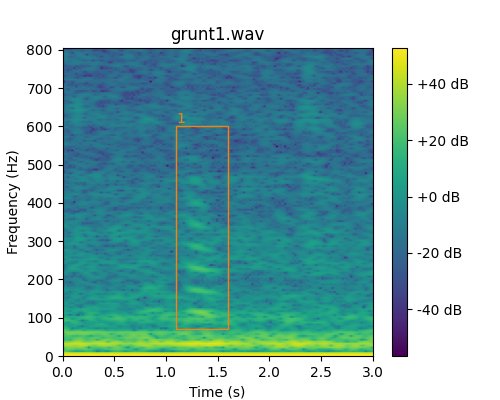`reduce_tonal_noise`(method='MEDIAN', **kwargs)[source]

Reduce continuous tonal noise produced by e.g. ships and slowly varying background noise

See `audio.image.reduce_tonal_noise()` for implementation details.

Currently, offers the following two methods:

1. MEDIAN: Subtracts from each row the median value of that row.

2. RUNNING_MEAN: Subtracts from each row the running mean of that row.

The running mean is computed according to the formula given in Baumgartner & Mussoline, JASA 129, 2889 (2011); doi: 10.1121/1.3562166

Args:
method: str

Options are ‘MEDIAN’ and ‘RUNNING_MEAN’

Optional args:
time_constant: float

Time constant in seconds, used for the computation of the running mean. Must be provided if the method ‘RUNNING_MEAN’ is chosen.

Example:
```>>> # read audio file
>>> from ketos.audio.waveform import Waveform
>>> aud = Waveform.from_wav('ketos/tests/assets/grunt1.wav')
>>> # compute the spectrogram
>>> from ketos.audio.spectrogram import MagSpectrogram
>>> spec = MagSpectrogram.from_waveform(aud, window=0.2, step=0.02)
>>> # keep only frequencies below 800 Hz
>>> spec = spec.crop(freq_max=800)
>>> # show spectrogram as is
>>> fig = spec.plot()
>>> fig.savefig("ketos/tests/assets/tmp/spec_before_tonal.png")
>>> plt.close(fig)
>>> # tonal noise reduction
>>> spec.reduce_tonal_noise()
>>> # show modified spectrogram
>>> fig = spec.plot()
>>> fig.savefig("ketos/tests/assets/tmp/spec_after_tonal.png")
>>> plt.close(fig)
```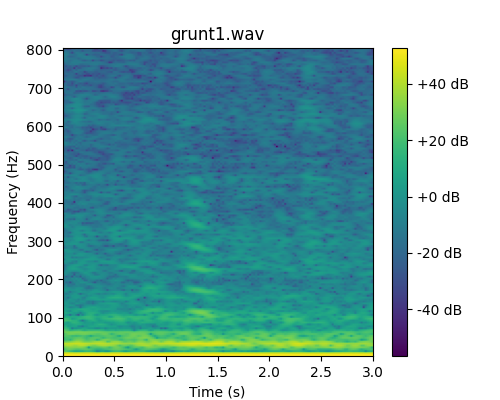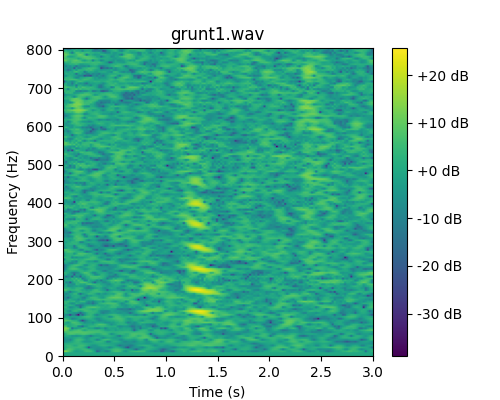`segment`(window, step=None)[source]

Divide the time axis into segments of uniform length, which may or may not be overlapping.

Window length and step size are converted to the nearest integer number of time steps.

If necessary, the spectrogram will be padded with zeros at the end to ensure that all segments have an equal number of samples.

Args:
window: float

Length of each segment in seconds.

step: float

Step size in seconds.

Returns:
specs: Spectrogram

Stacked spectrograms

`ketos.audio.spectrogram.``add_specs`(a, b, offset=0, scale=1, make_copy=False)[source]

Place two spectrograms on top of one another by adding their pixel values.

The spectrograms must be of the same type, and share the same time resolution.

The spectrograms must have consistent frequency axes. For linear frequency axes, this implies having the same resolution; for logarithmic axes with base 2, this implies having the same number of bins per octave minimum values that differ by a factor ofwhereis the number of bins per octave andis any integer. No check is made for the consistency of the frequency axes.

Note that the attributes filename, offset, and label of spectrogram b is being added are lost.

The sum spectrogram has the same dimensions (time x frequency) as spectrogram a.

Args:
a: Spectrogram

Spectrogram

b: Spectrogram

Spectrogram to be added

offset: float

Shift spectrogram b by this many seconds relative to spectrogram a.

scale: float

Scaling factor applied to signal that is added

make_copy: bool

Make copies of both spectrograms, leaving the orignal instances unchanged by the addition operation.

Returns:
ab: Spectrogram

Sum spectrogram

`ketos.audio.spectrogram.``load_audio_for_spec`(path, channel, rate, window, step, offset, duration, resample_method, id=None, normalize_wav=False, transforms=None)[source]

Load audio data from a wav file for the specific purpose of computing the spectrogram.

The loaded audio covers a time interval that extends slightly beyond that specified, [offset, offset+duration], as needed to compute the full spectrogram without zero padding at either end. If the lower/upper boundary of the time interval coincidences with the start/end of the audio file so that no more data is available, we pad with zeros to achieve the desired length.

Args:
path: str

Path to wav file

channel: int

Channel to read from. Only relevant for stereo recordings

rate: float

Desired sampling rate in Hz. If None, the original sampling rate will be used

window: float

Window size in seconds that will be used for computing the spectrogram

step: float

Step size in seconds that will be used for computing the spectrogram

offset: float

Start time of spectrogram in seconds, relative the start of the wav file.

duration: float

Length of spectrogrma in seconds.

resample_method: str
Resampling method. Only relevant if rate is specified. Options are
• kaiser_best

• kaiser_fast

• scipy (default)

• polyphase

See https://librosa.github.io/librosa/generated/librosa.core.resample.html for details on the individual methods.

id: str

Unique identifier (optional). If None, the filename will be used.

normalize_wav: bool

Normalize the waveform to have a mean of zero (mean=0) and a standard deviation of unity (std=1). Default is False.

Returns:
audio: Waveform

The audio signal

seg_args: tuple(int,int,int,int)

Input arguments for `audio.utils.misc.segment()`

`ketos.audio.spectrogram.``mag2mel`(img, num_fft, rate, num_filters, num_ceps, cep_lifter)[source]

Convert a Magnitude spectrogram to a Mel spectrogram.

Args:
img: numpy.array

Magnitude spectrogram image.

num_fft: int

Number of points used for the FFT.

rate: float

Sampling rate in Hz.

num_filters: int

The number of filters in the filter bank.

num_ceps: int

The number of Mel-frequency cepstrums.

cep_lifters: int

The number of cepstum filters.

Returns:
mel_spec: numpy.array

Mel spectrogram image

filter_banks: numpy.array

Filter banks

`ketos.audio.spectrogram.``mag2pow`(img, num_fft)[source]

Convert a Magnitude spectrogram to a Power spectrogram.

Args:
img: numpy.array

Magnitude spectrogram image.

num_fft: int

Number of points used for the FFT.

Returns:
: numpy.array

Power spectrogram image